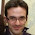## Wednesday, July 13, 2011

### Guarded recursion with a weaker-than-Nakano guard modality

We have a new draft paper up, on controlling the memory usage of FRP. I have to say that I really enjoy this line of work: there's a very strong interplay between theory and practice. For example, this paper --- which is chock full of type theory and denotational semantics --- is strongly motivated by questions that arose from thinking about how to make our implementation efficient.

In this post, I'm going to start spinning out some consequences of one minor point of our current draft, which we do not draw much attention to. (It's not really the point of the paper, and isn't really enough to be a paper on its own -- which makes it perfect for research blogging.) Namely, we have a new delay modality, which substantially differs from the original Nakano proposed in his LICS 2000 paper.

Recall that the delay modality $\bullet A$ is a type constructor for guarded recursion. I'll start by giving a small type theory for guarded recursion below.

$$\begin{array}{lcl} A & ::= & A \to B \;\;|\;\; \bullet A \;\;|\;\; \mathbb{N} \\ \Gamma & ::= & \cdot \;\;|\;\; \Gamma, x:A[i] \end{array}$$

As can be seen above, the types are delay types, functions, and natural numbers, and contexts come indexed with time indices $i$. So is the typing judgement $\Gamma \vdash e : A[i]$.

$$\begin{array}{ll} \frac{x:A[i] \in \Gamma \qquad i \leq j} {\Gamma \vdash x : A[j]} & \frac{\begin{array}{l} \Gamma \vdash e:A[i] & \Gamma,x:A[i] \vdash e' : B[i] \end{array}} {\Gamma \vdash \mathsf{let}\; x = e \;\mathsf{in}\; e' : B[i]} \\ & \\ \frac{\Gamma, x:A[i] \vdash e : B[i]} {\Gamma \vdash \lambda x.\;e : A \to B} & \frac{\begin{array}{l} \Gamma \vdash e : A \to B [i] & \Gamma \vdash e' : A[i] \end{array}} {\Gamma \vdash e \; e' : B[i]} \\ & \\ \frac{\Gamma \vdash e : A[i+1]} {\Gamma \vdash \bullet e : A[i]} & \frac{\begin{array}{ll} \Gamma \vdash e : \bullet A[i] & \Gamma, x:A[i+1] \vdash e' : B[i] \end{array}} {\Gamma \vdash \mathsf{let}\; \bullet x = e \;\mathsf{in}\; e' : B[i]} \\ & \\ \frac{} {\Gamma \vdash \mathsf{z} : \mathbb{N}[i]} & \frac{\Gamma \vdash e : \mathbb{N}[i+1]} {\Gamma \vdash \mathsf{s}(e) : \mathbb{N}[i]} \\ & \\ \frac{\Gamma, x:A[i+1] \vdash e : A[i]} {\Gamma \vdash \mu x.\;e : A[i]} & \frac{\begin{array}{l} \Gamma \vdash e : \mathbb{N}[i] \\ \Gamma \vdash e_1 : A[i] \\ \Gamma, x:\mathbb{N}[i+1] \vdash e_2 : A[i] \end{array}} {\Gamma \vdash \mathsf{case}(e, \mathsf{z} \to e_1, \mathsf{s}(x) \to e_2) : A[i]} \end{array}$$

The $i+1$ in the successor rule for natural numbers pairs with the rule for case statements, and this is what allows the fixed point rule to do its magic. Fixed points are only well-typed if the recursion variable occurs at a later time, and the case statement for numbers gives the variable one step later. So by typing we guarantee well-founded recursion!

The intro and elim rules for delays internalize increasing the time index, so that an intro for $\bullet A$ at time $i$ takes an expression of type $A$ at time $i+1$. We have a let-binding elimination form for delays, which differs from our earlier LICS paper, which had a direct-style elimination for delays. The new elimination is weaker than the old one, in that it cannot prove the isomorphism of $\bullet(A \to B) \simeq \bullet A \to \bullet B$.

This is really quite great, since that isomorphism was really hard to implement! (It was maybe half the complexity of the logical relation.) The only question is whether or not we can still give a categorical semantics to this language. In fact, we can, and I'll
describe it in my next post.

#### 3 comments:

1.I think I see an intro rule for delay, but it has ∙e:A[i] instead of e:∙A[i]? Is that just a typo (as it seems to be suggested by the text, and I'm tentatively assuming) or is that somehow intended?

1.This comment has been removed by the author.

2.The paper (now available at https://www.cl.cam.ac.uk/~nk480/popl074-krishnaswami.pdf) uses indeed ∙e:∙A[i], because ∙ is overloaded as a term constructor and a type constructor.